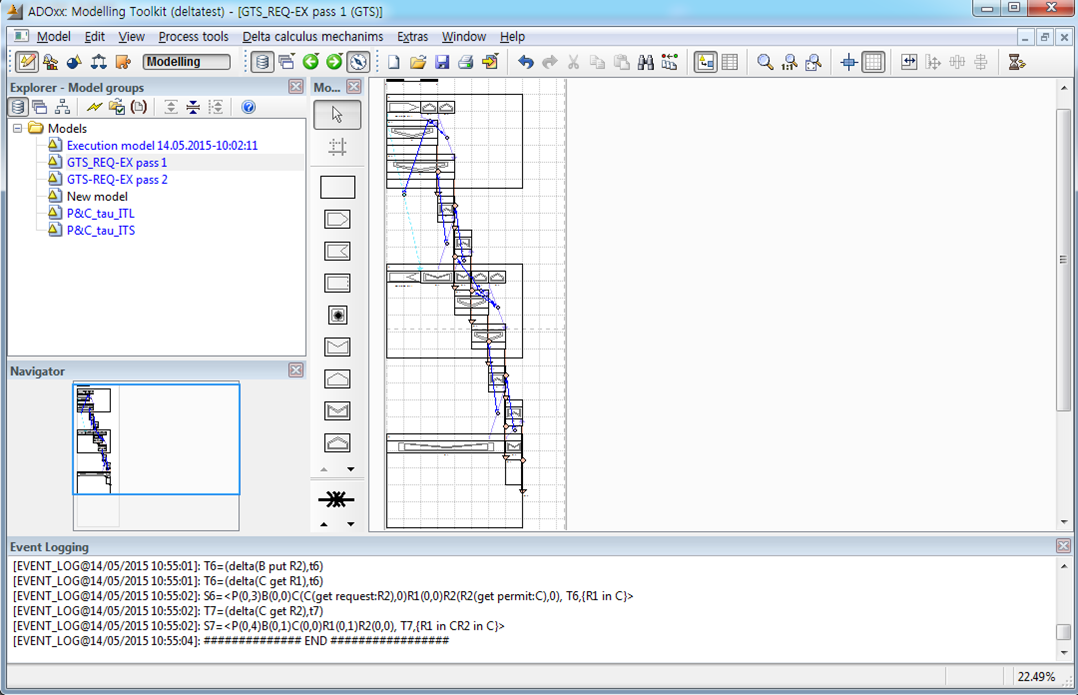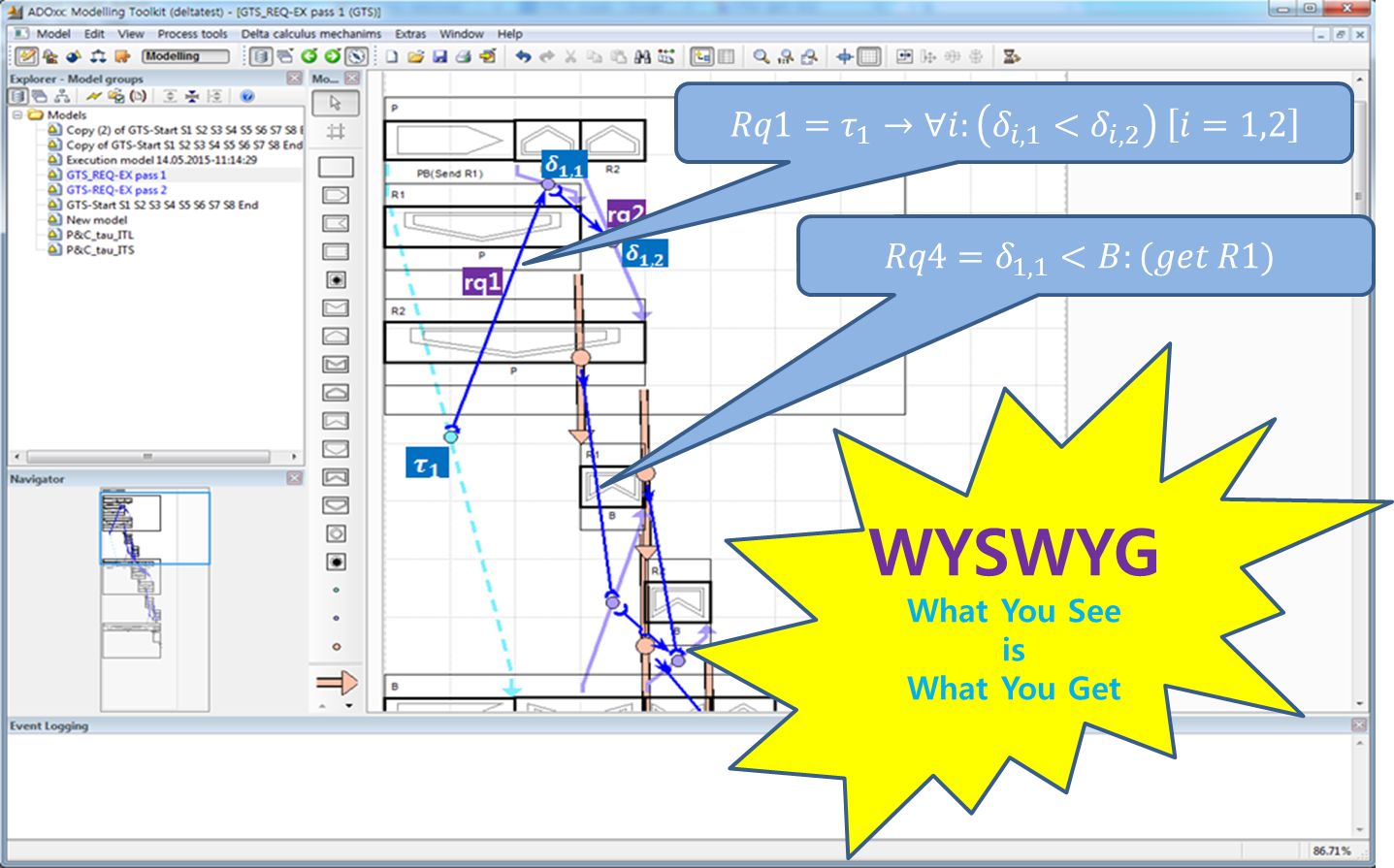1. Requirements

• Operational Requirements
• Producer produces two resources, R1 and R2.
• Produce stores the resources in Buffer in order.
• Producer informs Buffer of the order of R1 and R2, or R2 and R1.
• Consumer consumes the resources from Buffer in order.
• The order of the consumption is formed to Buffer by Consumer.
• Secure Requirements
• Security:
• The order should not be violated, since the first resource contains security information to decode the second resource.
• The propagation between the first and the second should be less than 30 seconds.
• Safety
• The resources produced by Producer should be consumed by Consumer less than 5 minutes.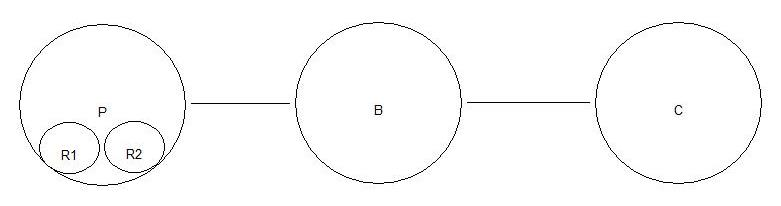2. δ-Calculus

• PBC=P[R1∥R2]∥B∥C
• P=(PB((Send R1) ̅ ).put R1.put R2+PB((Send R2) ̅ ).put R2.put R1).exit
• B=(PB(Send R1).get R1.get R2+PB(Send R2).get R2.get R2).put R1.put R2.exit
• C=get R1.get R2.exit
• R1=P put.B get.B put.C get.exit
• R2=P put.B get.B put.C get.exit

3. ITL View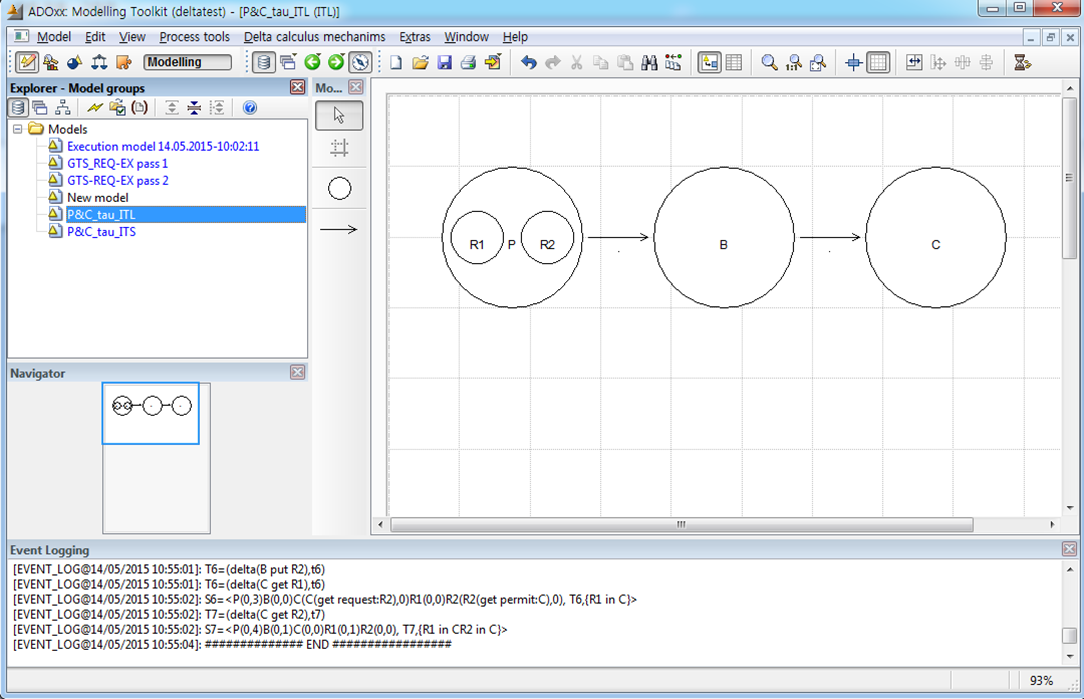4. ITS Views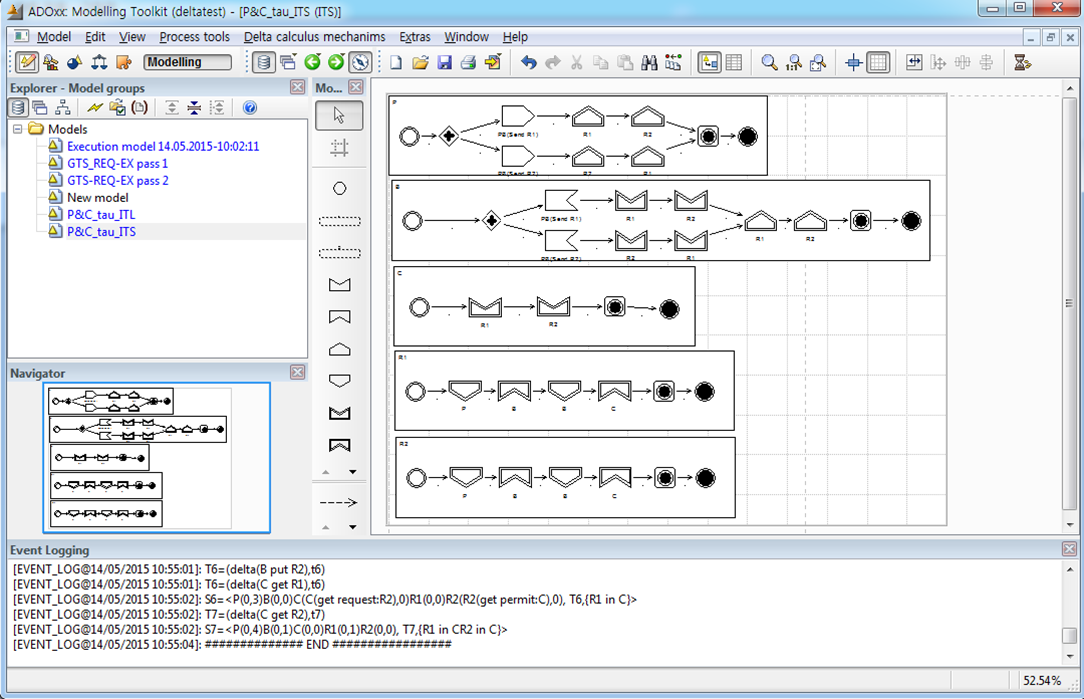5. Execution Models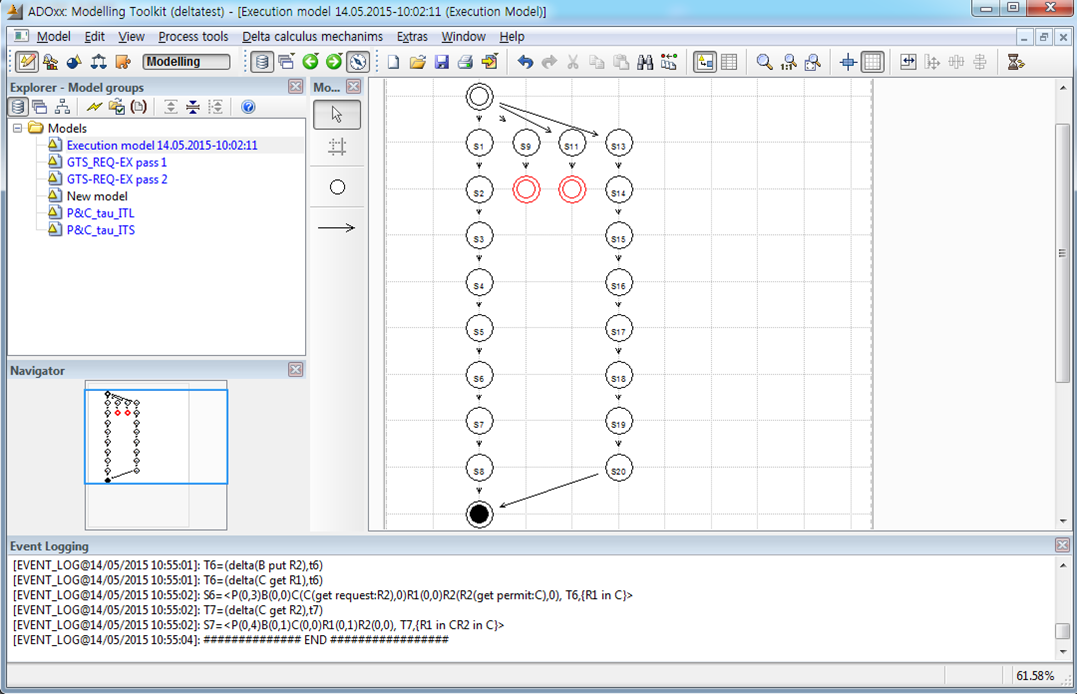6. Simulation7. GTS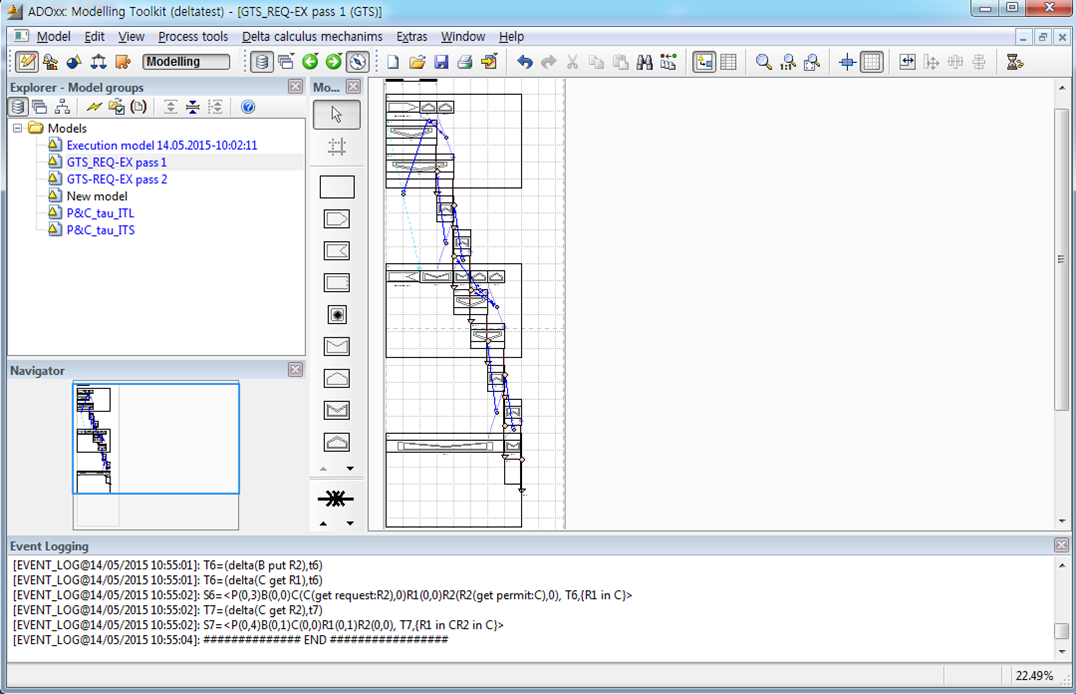8. GTS Logic

1) Interactions

• τ: Communication
• τ_1= (P:PB,((Send R1) ̅ ),B:PB(Send R1))
• τ_2=(P:PB,((Send R2) ̅ ),B:PB(Send R2))
• δ: Movements
• δ_1,1= (P:put R1,R1:P put)
• δ_1,2= (P:put R2,R2:P put)
• δ_2,1= (B:get R1,R1:B get)
• δ_2,2= (B:get R2,R2:P get)
• δ_3,1= (B:put R1,R1:B put)
• δ_3,2= (B:put R2,R2:B put)
• δ_4,1= (C:get R1,R1:C get)
• δ_4,2= (C:get R2,R2:C get)
2) Requirements
• Rq1=τ_1→∀i:(δ_(i,1)<δ_(i,2) )  [i=1,2]
• Rq2=τ_2→∀i:(δ_(i,2)<δ_(i,2)) [i=1,2]
• Rq3=τ_1τ_2<δ_1,1δ_1,2
• Rq4=δ_1,1<B:(get R1)
• Rq5=δ_1,2<B:(get R2)
• Rq6=δ_2,1<B:(put R1)
• Rq7=δ_2,2<B:(put R2)
• Rq8=δ_3,1<C:(get R1)
• Rq9=δ_3,2<C:(get R2)